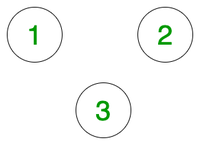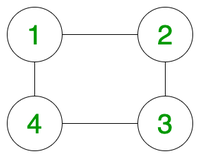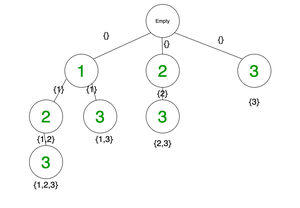# Maximal independent set from a given Graph using Backtracking

Given an undirected graph with V vertices and E edges, the task is to print all the independent sets and also find the maximal independent set(s).

Independent set is a set of vertices such that any two vertices in the set do not have a direct edge between them.

Maximal independent set is an independent set having highest number of vertices.

Note: There can be more than one independent and maximal independent sets for a given graph.

Examples:

Input:
V = 3, E = 0
Graph:Output:
{ }{ 1 }{ 1 2 }{ 1 2 3 }{ 1 3 }{ 2 }{ 2 3 }{ 3 }
{ 1 2 3 }
Explanation:
The first line represents all the possible indpendent sets for the given graph. The second line has the maximal independent sets possible for the given graph.

Input:
V = 4, E = 4
Graph:Output:
{ }{ 1 }{ 1 3 }{ 2 }{ 2 4 }{ 3 }{ 4 }
{ 1 3 }{ 2 4 }

## Recommended: Please try your approach on {IDE} first, before moving on to the solution.

Approach:
The idea is to use Backtracking to solve the problem. At every step, we need to check whether the current node has any direct edge with any of the nodes already present in our independent set. If not, we can add it to our independent set and recursively repeat the same process for all the nodes.

Illustration:
Recursion tree for the first example:In the above backtracking tree we will choose only those sets which are produced after adding a safe node to maintain the set as an independent set.

Below is the implementation of the above approach:

## CPP

 `// C++ Program to print the ` `// independent sets and ` `// maximal independent sets ` `// of the given graph ` ` `  `#include ` `using` `namespace` `std; ` ` `  `// To store all the independent ` `// sets of the graph ` `set > independentSets; ` ` `  `// To store all maximal independent ` `// sets in the graph ` `set > maximalIndependentSets; ` ` `  `map, ``int``> edges; ` `vector<``int``> vertices; ` ` `  `// Function to print all independent sets ` `void` `printAllIndependentSets() ` `{ ` `    ``for` `(``auto` `iter : independentSets) { ` `        ``cout << ``"{ "``; ` `        ``for` `(``auto` `iter2 : iter) { ` `            ``cout << iter2 << ``" "``; ` `        ``} ` `        ``cout << ``"}"``; ` `    ``} ` `    ``cout << endl; ` `} ` ` `  `// Function to extract all ` `// maximal independent sets ` `void` `printMaximalIndependentSets() ` `{ ` `    ``int` `maxCount = 0; ` `    ``int` `localCount = 0; ` `    ``for` `(``auto` `iter : independentSets) { ` ` `  `        ``localCount = 0; ` `        ``for` `(``auto` `iter2 : iter) { ` `            ``localCount++; ` `        ``} ` `        ``if` `(localCount > maxCount) ` `            ``maxCount = localCount; ` `    ``} ` `    ``for` `(``auto` `iter : independentSets) { ` ` `  `        ``localCount = 0; ` `        ``set<``int``> tempMaximalSet; ` ` `  `        ``for` `(``auto` `iter2 : iter) { ` `            ``localCount++; ` `            ``tempMaximalSet.insert(iter2); ` `        ``} ` `        ``if` `(localCount == maxCount) ` `            ``maximalIndependentSets ` `                ``.insert(tempMaximalSet); ` `    ``} ` `    ``for` `(``auto` `iter : maximalIndependentSets) { ` `        ``cout << ``"{ "``; ` `        ``for` `(``auto` `iter2 : iter) { ` `            ``cout << iter2 << ``" "``; ` `        ``} ` `        ``cout << ``"}"``; ` `    ``} ` `    ``cout << endl; ` `} ` ` `  `// Function to check if a ` `// node is safe node. ` `bool` `isSafeForIndependentSet( ` `    ``int` `vertex, ` `    ``set<``int``> tempSolutionSet) ` `{ ` `    ``for` `(``auto` `iter : tempSolutionSet) { ` `        ``if` `(edges[make_pair(iter, vertex)]) { ` `            ``return` `false``; ` `        ``} ` `    ``} ` `    ``return` `true``; ` `} ` ` `  `// Recursive function to find ` `// all independent sets ` `void` `findAllIndependentSets( ` `    ``int` `currV, ` `    ``int` `setSize, ` `    ``set<``int``> tempSolutionSet) ` `{ ` `    ``for` `(``int` `i = currV; i <= setSize; i++) { ` `        ``if` `(isSafeForIndependentSet( ` `                ``vertices[i - 1], ` `                ``tempSolutionSet)) { ` `            ``tempSolutionSet ` `                ``.insert(vertices[i - 1]); ` `            ``findAllIndependentSets( ` `                ``i + 1, ` `                ``setSize, ` `                ``tempSolutionSet); ` `            ``tempSolutionSet ` `                ``.erase(vertices[i - 1]); ` `        ``} ` `    ``} ` `    ``independentSets ` `        ``.insert(tempSolutionSet); ` `} ` ` `  `// Driver Program ` `int` `main() ` `{ ` `    ``int` `V = 3, E = 0; ` ` `  `    ``for` `(``int` `i = 1; i <= V; i++) ` `        ``vertices.push_back(i); ` ` `  `    ``vector > inputEdges; ` ` `  `    ``pair<``int``, ``int``> edge; ` `    ``int` `x, y; ` `    ``for` `(``int` `i = 0; i < E; i++) { ` `        ``edge.first = inputEdges[i].first; ` `        ``edge.second = inputEdges[i].second; ` `        ``edges[edge] = 1; ` `        ``int` `t = edge.first; ` `        ``edge.first = edge.second; ` `        ``edge.second = t; ` `        ``edges[edge] = 1; ` `    ``} ` ` `  `    ``set<``int``> tempSolutionSet; ` ` `  `    ``findAllIndependentSets(1, ` `                           ``V, ` `                           ``tempSolutionSet); ` ` `  `    ``printAllIndependentSets(); ` ` `  `    ``printMaximalIndependentSets(); ` ` `  `    ``return` `0; ` `} `

Output:

```{ }{ 1 }{ 1 2 }{ 1 2 3 }{ 1 3 }{ 2 }{ 2 3 }{ 3 }
{ 1 2 3 }
```My Personal Notes arrow_drop_upCheck out this Author's contributed articles.

If you like GeeksforGeeks and would like to contribute, you can also write an article using contribute.geeksforgeeks.org or mail your article to contribute@geeksforgeeks.org. See your article appearing on the GeeksforGeeks main page and help other Geeks.

Please Improve this article if you find anything incorrect by clicking on the "Improve Article" button below.